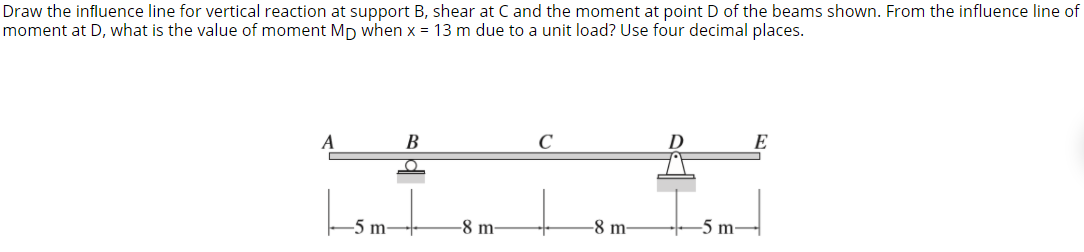# Question Draw the influence line for vertical reaction at support B, shear at C and the moment at point of the beams shown. From the influence line of moment at D, what is the value of moment Mp when x = 13 m due to a unit load? Use four decimal places. A B с D E O -5 m -8 m -8 m -5 m5H3AM4 The Asker · Civil EngineeringTranscribed Image Text: Draw the influence line for vertical reaction at support B, shear at C and the moment at point of the beams shown. From the influence line of moment at D, what is the value of moment Mp when x = 13 m due to a unit load? Use four decimal places. A B с D E O -5 m -8 m -8 m -5 m
More
Transcribed Image Text: Draw the influence line for vertical reaction at support B, shear at C and the moment at point of the beams shown. From the influence line of moment at D, what is the value of moment Mp when x = 13 m due to a unit load? Use four decimal places. A B с D E O -5 m -8 m -8 m -5 m